Next: Numerical Experiments Up: Simple stellar models and Previous: The TOV equations   Contents

## Implementation of the code

As in the case of the geodesic equations we need to cast our system as first order form. First we solved the EOS (24) for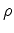,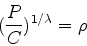(36)

Then we use this equation in the preceeding hydrostatic equilibrium equations to get the following system of first order equations,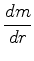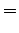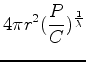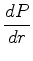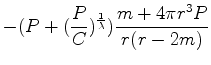(37)

The equations are coded in the subroutine tt fcn of LSODA, as in the case of the geodesic motion. As a precautory measure we have a lower bound on the pressure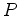to zero, so we avoid problems with the integration. for the initial conditions we provide the program, tt star.f, with an initial central pressure and central mass. The central mass is zero initially, to avoid a singularity in the origin, and we calculate the initial central presure using equation (37), and some initlal central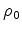, so the implementation of the equations is:
c        Initial central density

rho = (DABS(P/C))**(1.d0/lambda)

ydot(1) = 4.d0*Pi*r**2*rho

c        lower bound to zero
if ( r .le. 0.d0 ) then
phidot = 0.0
else
phidot = (m+4.d0*Pi*r**3*P)/(r*(r-2.d0*m))
endif

ydot(2) = -(P+rho)*phidot

At every step we calculate a new radius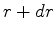, then calculateusing the equation of state,and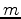. We detected another problem when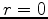for (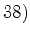. To set an initial condition for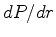we have to analyze the limit when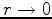. Both the first and second derivatives are finite in this limit, so we can use this value as an initial condotion for.

The usage of the program is the following:

 usage:  star <rho_0> <C> <lambda> <dr>
c================================================================
c     Command line info
c
c     <rho_0>   central density of the compact object
c
c     <C>       Constant in the equation of state
c
c     <lambda>  exponent of the density in the equation of state.
c
c     <dr>      integration step increment in radius.
c================================================================

As a suggestion from the instructor it delivers the logarithm in base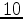of the central density and the mass or the radius.

To perform the survey of the phase space we need the values of mass and radius at different central densities. With this objetive we wrote a code denominated survey.f that uses star.f as a subroutine, integra.f.

lnx1 111> ./survey
Phys 555 Numerical Relativity Group 2
usage:  survey <rhomax> <deltarho> <C>
<lambda> <dr> <tol>
c===========================================================
c     Physics 555b Numerical Relativity Group 2
c
c     survey.f
c     This program goes thru different values of the
c     central density so we can plot M vs rho0, and radius
c     vs rho0. The integration of the equations is carried
c     out by the subroutine integra.
c     April-March 2205
c
c     Command line info
c
c     <rho_0_up> for the upper limit of the central density
c
c     <deltarho> for the setp increment in rho_0
c
c     <C>  Constant in the Polytropic equation of state
c
c     <lambda>   exponent of rho in equation of state
c
c     <dr>       integration step increment of radius
c===========================================================Next: Numerical Experiments Up: Simple stellar models and Previous: The TOV equations   Contents
Benjamin Gutierrez 2005-07-23